Essential Concept 7: Autoregressive (AR) Models | IFT World
101 Concepts for the Level I Exam

# Essential Concept 7: Autoregressive (AR) ModelsAn autoregressive time series model is a linear model that predicts its current value using its most recent past value as the independent variable. An AR model of order p, denoted by AR(p) uses p lags of a time series to predict its current value.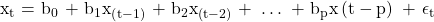The chain rule of forecasting is used to predict successive forecasts.
The one-period ahead forecast of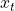from an AR(1) model is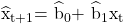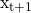can be used to forecast the two-period ahead value :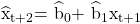LIVE WEEKEND CLASSES *For 2024 Exam candidates*
This is default text for notification bar